# Drawing functions in ggplot2 with geom_function

## Plotting functions in ggplot2

The `geom_function` can be used to draw functions in ggplot2. In this scenario you don’t need to pass a data frame to `ggplot`, but to specify the axis limits with `xlim` and the function to be plotted.

``````# install.packages("ggplot2")
library(ggplot2)

ggplot() +
xlim(c(0, 5)) +
geom_function(fun = cos)``````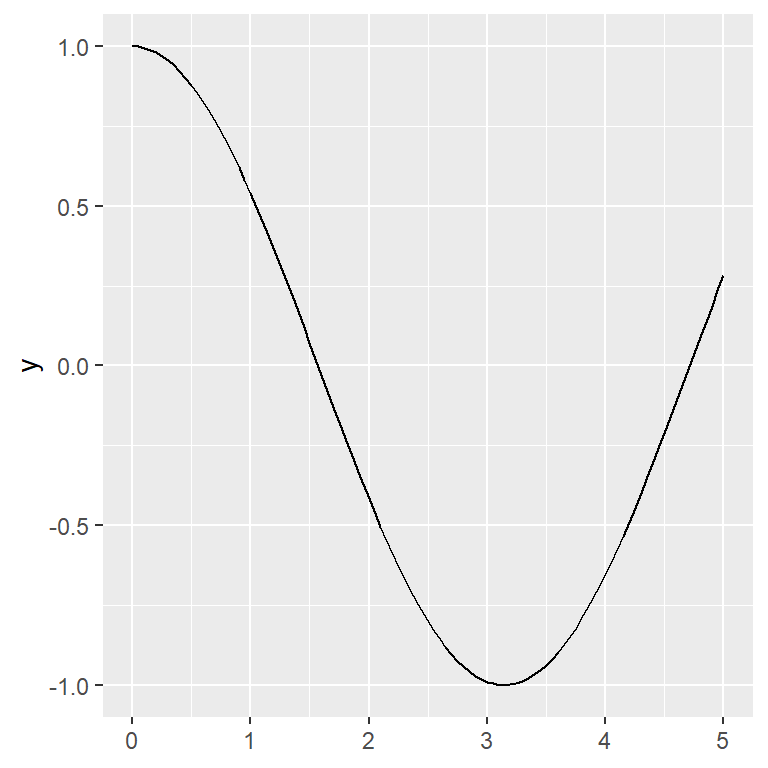Line customization

The function drawn can be customized with the typical arguments, such as `color`, `lwd` and `linetype` for the color, line width and line type, respectively.

``````# install.packages("ggplot2")
library(ggplot2)

ggplot() +
xlim(c(0, 5)) +
geom_function(fun = cos,
colour = "red",
lwd = 1,
linetype = 1)``````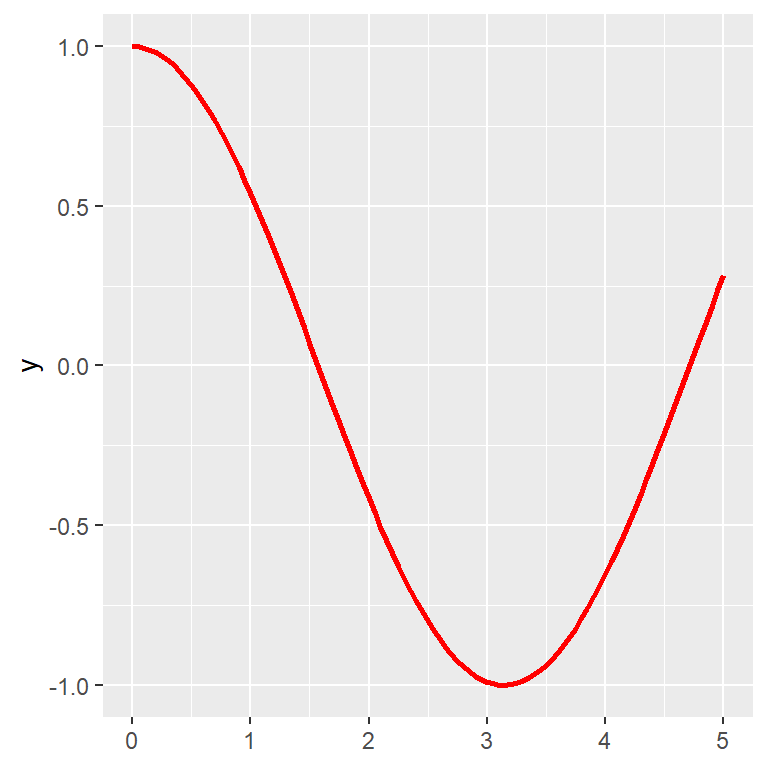stat_function

You can also use `stat_function` instead of `geom_function`. In this scenario you can change the default geom, as shown in the example below.

``````# install.packages("ggplot2")
library(ggplot2)

ggplot() +
xlim(c(0, 5)) +
stat_function(fun = cos,
geom = "point")``````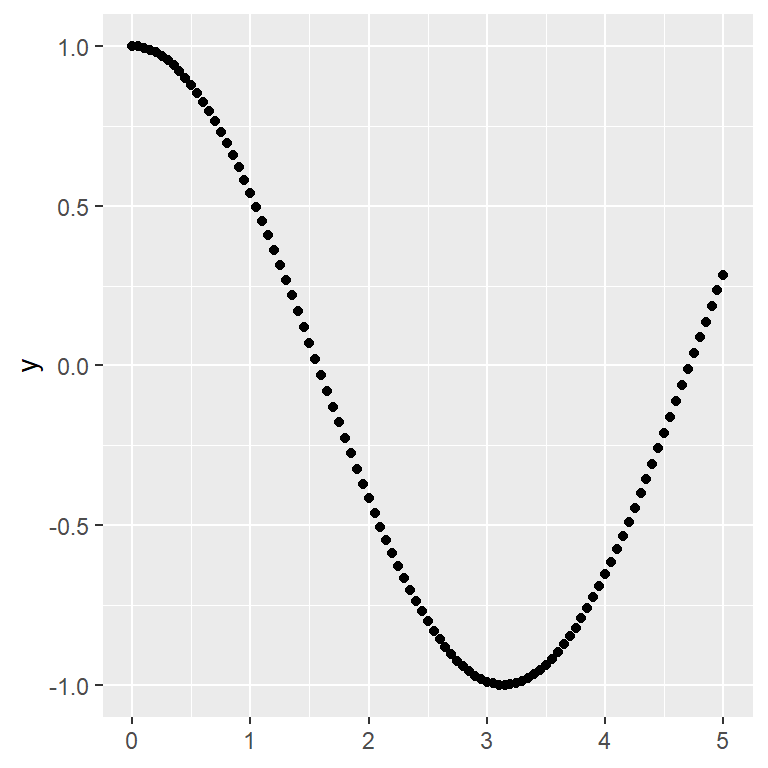Note that if the function you are plotting has additional arguments you can pass them through a list with the `args` argument. In the following example we are plotting a bessel function with `besselJ`, which requires an argument named `nu`.

``````# install.packages("ggplot2")
library(ggplot2)

ggplot() +
xlim(c(0, 50)) +
geom_function(fun = besselJ,
args = list(nu = 2))``````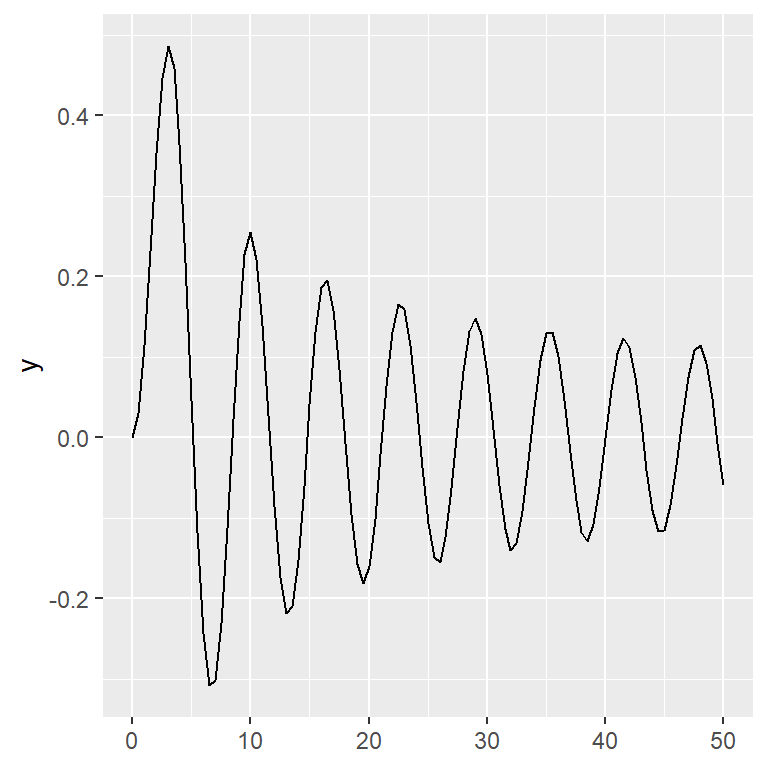Number of points

It should be noted that the number of points used to draw the function might not be enough, as in the previous example. To create a smoother function you can increase the value of the argument `n`.

``````# install.packages("ggplot2")
library(ggplot2)

ggplot() +
xlim(c(0, 50)) +
geom_function(fun = besselJ,
n = 400,
args = list(nu = 2))``````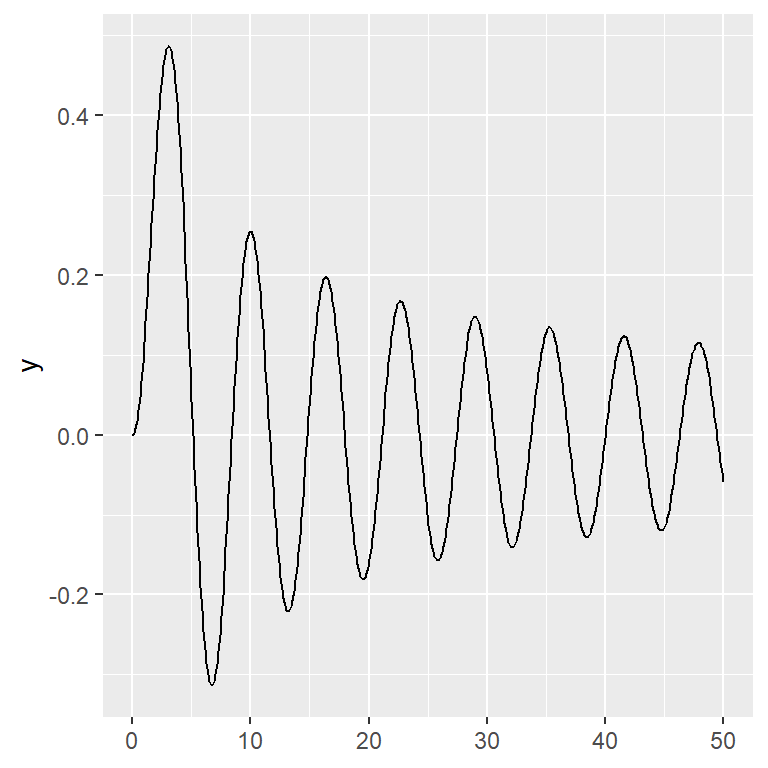## Overlaying several functions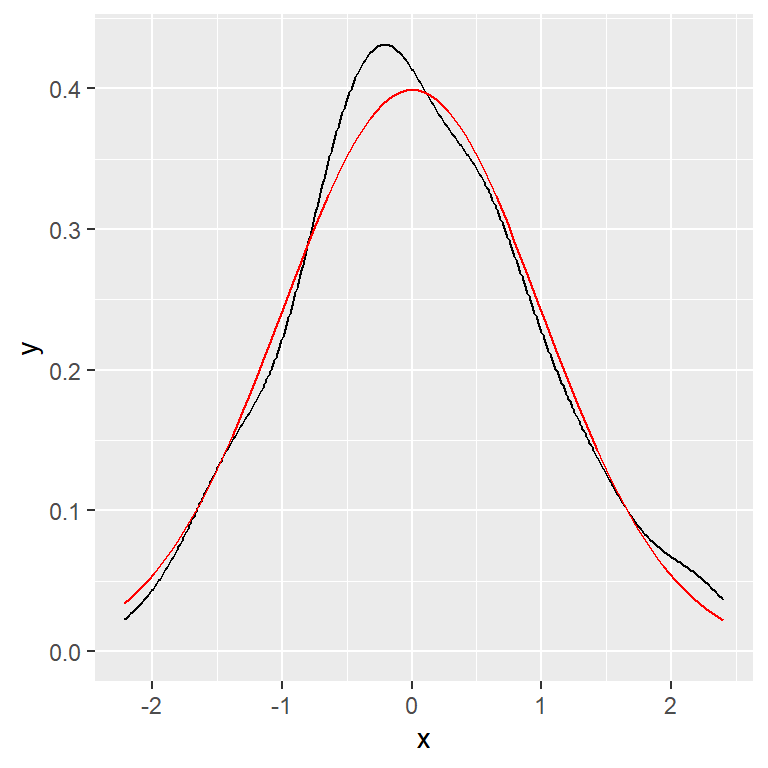Overlay over existing plot

If you have created a plot you can add a function over it with `geom_function`. The following example overlays the theoretical normal distribution over a normal distribution generated with 200 samples.

``````# install.packages("ggplot2")
library(ggplot2)

set.seed(1)
df <- data.frame(x = rnorm(200))

ggplot(df, aes(x = x)) +
geom_density() +
geom_function(fun = dnorm, colour = "red")``````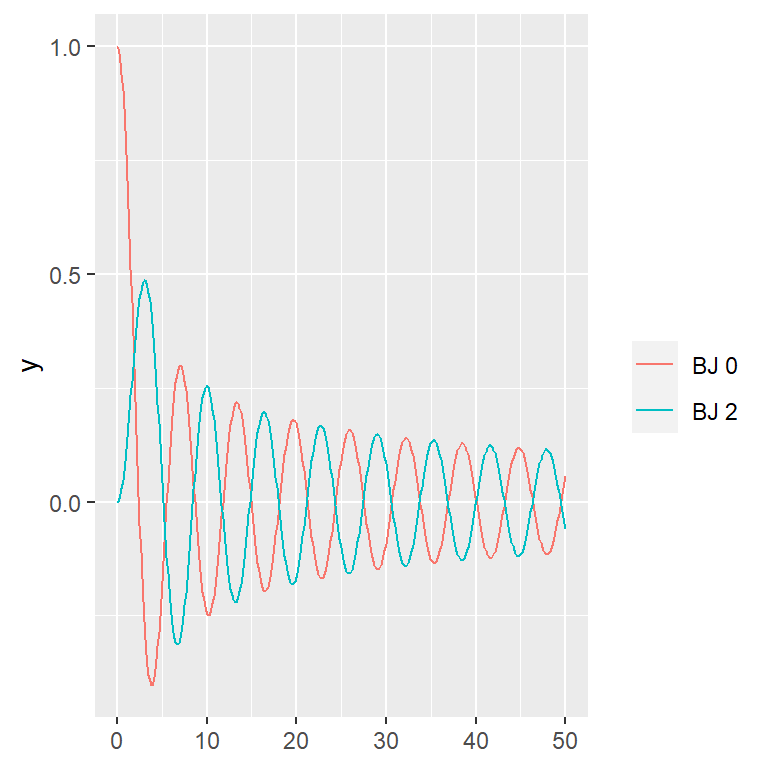Finally, it is worth to mention that you can add several functions to the same plot, adding more layers.

``````# install.packages("ggplot2")
library(ggplot2)

ggplot() +
xlim(c(0, 50)) +
geom_function(fun = besselJ, n = 400,
aes(color = "BJ 0"),
args = list(nu = 0)) +
geom_function(fun = besselJ, n = 400,
aes(color = "BJ 2"),
args = list(nu = 2)) +
guides(colour = guide_legend(title = ""))``````